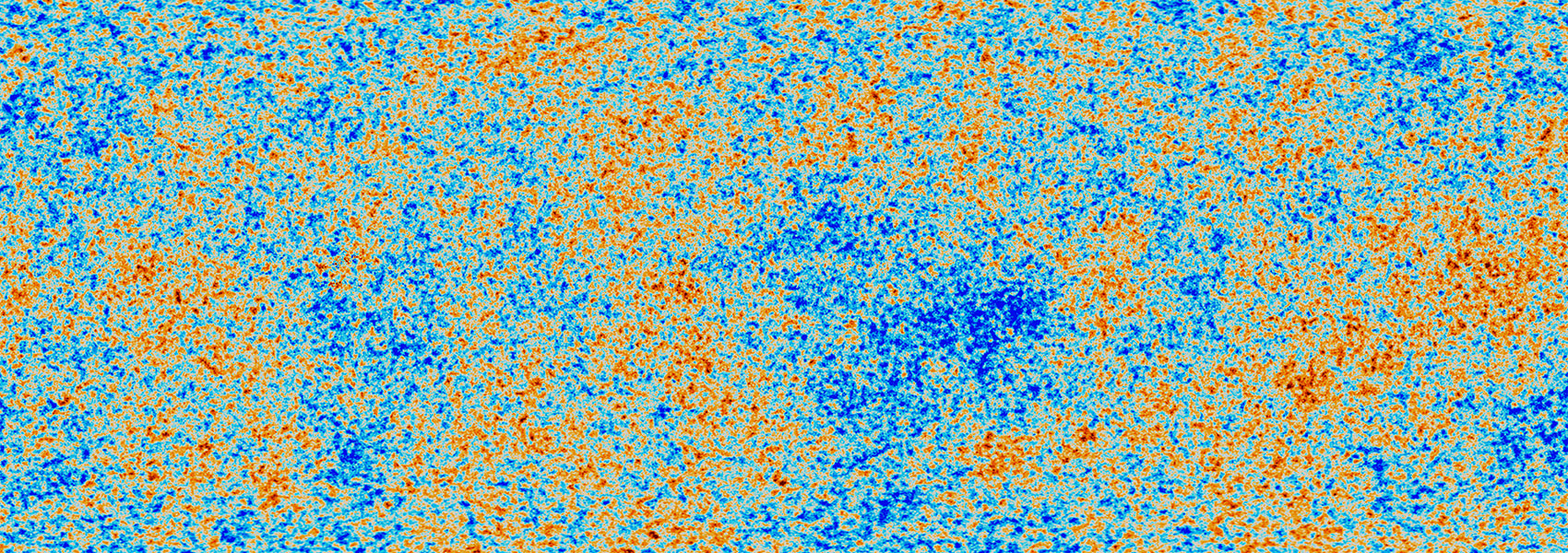# Erratum: “Understanding The Effects Of Stellar Multiplicity On The Derived Planet Radii From Transit Surveys: Implications for Kepler, K2, and TESS” (ApJ, 805, 16, )

January 2017 • 2017ApJ...834...96C

Authors • Ciardi, David R. • Beichman, Charles A. • Horch, Elliott P. • Howell, Steve B.

Abstract • There was an error in the published version of Equation (6); the equation should read as The calculations and results presented in the paper utilized the correct form of the equation and are unaffected by the error in the equation. The authors sincerely regret the error.